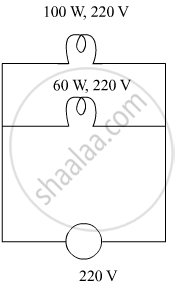# Two electric bulbs rated 100 W; 220 V and 60 W; 220 V are connected in parallel to electric mains of 220 V. Find the current drawn by the bulbs from the mains. - Science

Sum

Two electric bulbs rated 100 W; 220 V and 60 W; 220 V are connected in parallel to electric mains of 220 V. Find the current drawn by the bulbs from the mains.

#### SolutionFirstly calculating the resistance of the electric bulbs,

P="V"^2/"R"

⇒ "R"="V"^2/"P"

⇒ "R"_1 =220^2/100 =484 Ω

⇒ "R"_2=220^2/60=2420/3 Ω

"R"_"eq" = ("R"_1"R"_2)/("R"_1+"R"_2)=(484xx(2420//3))/(484+(2420//3))=302.5Ω

Total current drawn by the bulbs from the mains supply,
"I"=220/302.5=0.73 "A"

Concept: Electric Current
Is there an error in this question or solution?